# NCERT Solutions for Class 7 Maths Chapter 6 The Triangle and its Properties (EX 6.1) Exercise 6.1

## NCERT Solutions for Class 7 Maths Chapter 6 The Triangle and its Properties (EX 6.1) Exercise 6.1

NCERT Solutions for Class 7 Maths Chapter 6 - The Triangle and its Properties - Exercise 6.1 is an effective study material for students aiming to score high marks. The Class 7 Maths Ex 6.1 solutions are curated by some of the best Maths tutors of the country. These notes are ideal for studying as well as for practise. You can start your preparation by simply downloading the NCERT Maths Class 7 Exercise 6.1 Solution PDF. Register Online for NCERT Solutions Class 7 Science tuition on Vedantu.com to score more marks in CBSE board examination. Students can also download NCERT Solution PDF for all subjects to prepare for their forthcoming Exams.

Do you need help with your Homework? Are you preparing for Exams?
Study without Internet (Offline)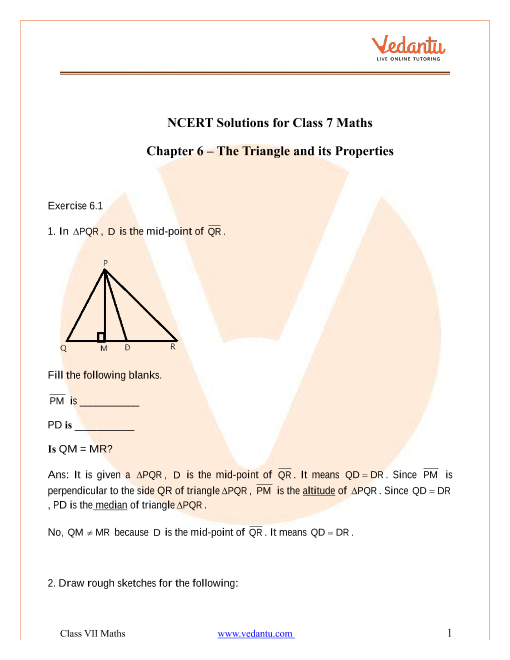Book your Free Demo session
Get a flavour of LIVE classes here at Vedantu## Access NCERT Solutions for Class 7 Maths Chapter 6 – Triangles and its properties

Exercise 6.1

Refer to page 3 for exercise 6.1 in the PDF.

1. In $\vartriangle PQR$, $D$ is the mid-point of $\overline {QR}$.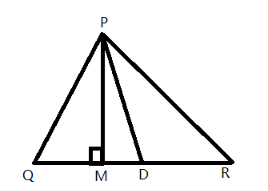Fill the following blanks.

$\overline {PM}$ is ___________ and $PD$ is _______. Is $QM = MR$?

Ans: It is given a $\vartriangle PQR$, $D$ is the mid-point of $\ overline {QR}$. It means $QD = DR$. Since $\overline {PM}$ is perpendicular to the side $QR$ of triangle $\vartriangle PQR$, $\overline {PM}$ is the altitude of  $\vartriangle PQR$. Since $QD = DR$, $PD$ is the median of triangle $\vartriangle PQR$.

No, $QM \ne MR$ because $D$ is the mid-point of $\overline {QR}$. It means $QD = DR$.

2. Draw rough sketches for the following:

a) Draw a rough sketch of $\vartriangle ABC$, where $BE$ is a median.

Ans: A median of a triangle is a line segment that is drawn from a vertex to the opposite side of the vertex and it divides the opposite side into two equal parts. The rough sketch of $\vartriangle ABC$, where $BE$ is a median, is drawn below.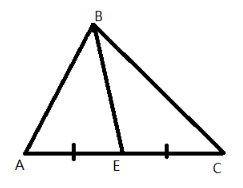Here $BE$ is a median in $\vartriangle ABC$ and $AE = EC$.

b) Draw rough sketches of $\vartriangle PQR$, where $PQ$ and $PR$ are the altitudes of the triangle.

Ans: An altitude of a triangle is defined as a perpendicular drawn from the vertex to the line containing the opposite side of the triangle. The rough sketch of $\vartriangle PQR$, where $PQ$ and $PR$ are the altitudes of the triangle is drawn below.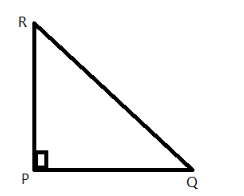c) Draw rough sketches of $\vartriangle XYZ$, where $YL$ is an altitude in the exterior of the triangle.

Ans: An altitude of a triangle is defined as a perpendicular drawn from the vertex to the line containing the opposite side of the triangle. The rough sketch of $\vartriangle XYZ$, $YL$ is an altitude in the exterior of the triangle is drawn below.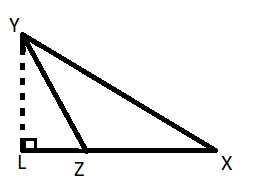3. Verify by drawing a diagram if the median and altitude of an isosceles triangle can be the same.

Ans: It is given an isosceles triangle. It is required to verify if the median and altitude of the given triangle can be the same. To do this, construct an isosceles triangle. An isosceles triangle has two equal sides.

Construct an isosceles triangle $\vartriangle ABC$ with sides$AB = AC$ and draw a median $AL$ that divides the base of the triangle into two equal parts.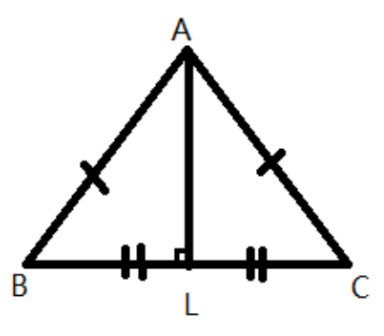From the triangle, it can be seen that the median makes a ${90^ \circ }$ angle with the base $BC$. So, $AL$ is the altitude of the triangle $\vartriangle ABC$. Hence verified, $AL$ is the median and altitude of the given triangle $\vartriangle ABC$.

## CBSE Solutions for Class 7 Maths Chapter 6 - The Triangle and its Properties Exercise 6.1

The Maths Class 7 Chapter 6 Exercise 6.1 is prepared by the best subject experts with more than a decade of teaching experience. These experienced teachers bring a lot to the table. They know the importance of every section and they have prepared the solution notes accordingly. They are subject-matter experts and they know the importance of this particular chapter. Furthermore, years of experience have made them well acquainted with the examiner's psychology. As a result, the answers in these solutions help you to score high marks. All the essential segments are covered beautifully in these notes. This NCERT Maths Solution Class 7 Chapter 6 Exercise 6.1 is prepared as per the latest NCERT guidelines.

Triangle and its properties is a very crucial chapter which has limitless implications. This Chapter will form an integral part of your Maths syllabus on the higher classes. In this NCERT Solutions Class 7 Maths Ex 6.1, a special focus is garnished on one of the most important topics of Geometry - Pythagoras Theorem.

### What is Pythagoras' Theorem?

This forms the basis of Geometry. The application of this Theorem is vast and you need to master this Theorem completely. Also known as the Pythagorean Theorem, this is one of the most important mathematical formulas and it is indeed the heartbeat of Geometry. This best known mathematical formula portrays the relationship between the 3 sides (Perpendicular, Base, and Hypotenuse) of a right-angle triangle. The Hypotenuse is the longest side of the triangle. When it comes to Triangle and its Properties, you need to have a clear knowledge about this all-important Theorem.

Vedantu's professional experts realise the importance of this segment. This is a big reason why a lot of impetus is given in this particular section. All the problems are broken down and are properly explained with relevant examples for easy and simple learning. In this Class 7th Maths Chapter 6 Exercise 6.1, you will learn about all the important segments without a hassle. This is what smart learning is all about. For a crucial segment such as this, conceptual understanding is more important than anything else. This is why all the parts are covered in this note along with detailed explanations for a better understanding and a stronger base.

There are no rewards for guessing the fact that Class 7 Maths syllabus is completely conceptual and there are a myriad of things to cover. At this stage, fresh things are introduced on every page. There are unending things to cover but you only have limited time. This is where revision notes such as NCERT Solution for Class 7 Maths Chapter 6 Exercise 6.1 is so helpful. It explains the concepts and also helps you in practice. This is exactly what you need to score big on your upcoming exams.

### Score Big with NCERT Solution for Class 7 Maths Chapter 6 Exercise 6.1

When it comes to  CBSE Class 7 Maths syllabus, the first thing that comes to mind is Triangle and its Properties. This is undoubtedly one of the most crucial chapters in the syllabus. This Chapter will build your base which will play a key role in the higher classes. Triangle and its Properties will put your base and basic understanding skills at test. This is exactly why one of the main objectives was to step-by-step break down all the crucial segments in this Chapter to make the concepts clear and facilitate smart learning. The NCERT Solutions for Class 7 Maths Chapter 6 Ex 6.1 lays a special focus on making the concepts clear with comprehensive explanations aided with simple solutions. With this note, you will feel more confident as your concepts will be clear helping you to solve all types of problems related to this very important Chapter. Once you understand the framework, the application is going to be easy. However, regular practice should be a mandate at this stage. Unlike other subjects like Social Studies or English, Maths is something that you should practice once every day because it is entirely conceptual. However, there are so many chapters and you cannot practice all at a time.  This is where study materials like NCERT Solution for Class 7 Maths Exercise 6.1 will help you. No need to go through the unending pages of the textbook. Just study the PDF from your smartphone.

### Some Useful Education Tips to Score Good Marks in Your Upcoming Maths Exam

For a subject like Maths, you need to practice, understand,  and concentrate  Maths is one of the most feared subjects. Mathematical anxiety is very common among Class 7 students. This phobia arises due to lack of understanding.

The best way to combat anxiety related to this subject is by understanding it. Maths is an extremely interesting subject and is also very scoring if you can understand the concepts, ideas, and applications.

How to do that?

Here are some key tips that will certainly help you to master this subject:

• Plan Your Day- The CBSE Class 7 syllabus is quite vast with a plethora of aspects. However, you cannot afford to score excellently in a particular chapter. As a result, you cannot dedicate an entire day in practising Triangle and its Properties or Ratio and Proportion. This is why you need to segregate the day into various parts dedicated to diverse chapters. Make the most of the available time.

• Understand Before Practice- Maths is a subject which requires diligent practice. You can definitely bag excellent marks with a clear concept. This is why understanding not learning should be the initial focus point. The Maths NCERT Solution Class 7 Chapter 6 Exercise 6.1 is solely focused on clearing your concepts for a better understanding. A clear concept can certainly do wonders.

• Practice Matters-  Regular practise should be the main focus. You can practice the same sums again and again but every time you will be learning something new. This is why the importance of practice is second to none when it comes to a concept-based chapter such as Triangle and its Properties. Consult the NCERT Solutions Class 7 Maths Chapter 6 Exercise 6.1 every time you get stuck.

### Why Vedantu?

Vedantu has been the pioneer of Indian education by transforming the education system with smart learning. Vedantu is the country's number 1 educational platform consisting of veteran teachers. Vedantu is completely reliant on a student-centred approach and this is one of the biggest reasons behind their immense success. Today, this educational platform is behind the success stories of thousands of students who have cleared various exams in flying colours.

From Maths to Political Science, from Physics to Geography, this online education platform is jam-packed with subject-matter experts. Just like this NCERT Class 7 Maths Exercise 6.1 revision solution PDF, all the notes are prepared only by subject-matter veterans. They have years of expertise in this field and they have graduated from some of the most recognized international universities. They have so many accolades. Learning from such reputed teachers has been a dream of millions. Vedantu fulfils this dream, and that too without any fee or expense. Vedantu's platform connects the students to some of the excellent teachers. The main objective is to help all the students in the country to achieve their goal.

You can start by just downloading the official Vedantu app or by bookmarking the official Vedantu site. Register yourself for the webinars conducted by some renowned subject-matter experts and stand out from the rest. This is all you need to score great marks in your upcoming papers.SHARETWEETSHARESUBSCRIBE